Like   Tweet   Pin   +1   in

## Cornerstone Conference is a body of IPHC Churches existing to equip ministers and churches to fulfill the Great Commission!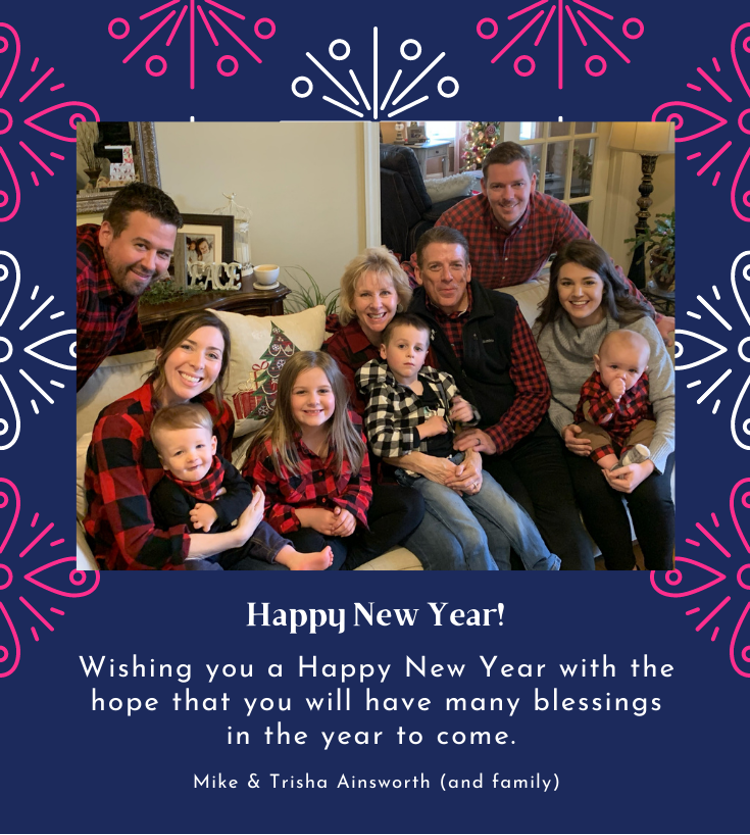table div table+table+table+table+table div table{width:100%;padding:0}table div table+table+table+table+table div table img{width:96.23%;padding:0;float:none}table div table+table+table+table+table div table td{width:100%;padding:0 1.88% 18px}/* styles */table div table+table+table+table+table+table+table div table{width:100%;padding:0}table div table+table+table+table+table+table+table div table img{width:96.23%;padding:0;float:none}table div table+table+table+table+table+table+table div table td{width:100%;padding:0 1.88% 18px}/* styles */# Happy New Year!

## By D. Stuart Briscoe

 /* styles */ Have you ever wondered how many seconds in this year you'll be unhappy? Have you ever thought how much can happen in a second? When you say, "Happy New Year!" you're saying a remarkable thing. If we're going to talk about having a happy new year, there are a few things we need to bear in mind. We’re not always sure what happiness is. For a lot of people, happiness depends on their happenings. If their happenings don't happen the way they want them to happen, they're unhappy! Some people spend their time organizing their happenings to make sure everything happens the way they want it to happen. The assumption is this: if they can make everything happen the way they want, they'll be happy. There are two problems with that: you can't do it; even if you could, you'd probably be bored. Alexander the Great got everything his way. He conquered everything and then sat down to cry, because he was so young and there was nothing left to conquer. For people who get everything they want, life is good. They have everything, and they don't know what to do with it. The Greeks had a word for happiness: makarios. This word described what they perceived as being the experience of the gods. The Greeks had lots of gods, and the gods were sort of magnified human beings; they had all the failings of human beings and all the strengths. For Greeks, the idea of the gods was that they had everything made. The word makarios found its way into the New Testament, and it is translated "blessed" or "happy." Jesus picked up on this word, and said some stuff that will absolutely blow your mind. Listen to what he said: Blessed are the poor in spirit. Blessed are those who mourn. Blessed are the meek. Blessed are those who hunger and thirst for righteousness. Blessed are the merciful. Blessed are the pure in heart. Blessed are the peacemakers. Blessed are those who are persecuted because of righteousness. Jesus is saying that happiness, or makarios—having everything just wonderful—comes not from having everything; it can come through being poor, through mourning, through hungering, through thirsting. It can come through being persecuted for righteousness' sake. That's exactly the opposite of what we think is the road to happiness. So Happy New Year! But remember two things. Define happiness correctly. Happiness is not just getting all your happenings to happen the way you want them to happen. Secondly, make certain you're thinking through all the possibilities of this year. You've got to reckon that you may not always be able to control them. There's a time for everything. With that in mind, let's turn to a passage from Ecclesiastes. "There's a time for everything and a season for every activity under heaven: a time to be born and a time to die, a time to plant and a time to uproot, a time to kill and a time to heal, a time to tear down and a time to build, a time to weep and a time to laugh, a time to mourn and a time to dance, a time to scatter stones and a time to gather them, a time to embrace and a time to refrain, a time to search and a time to give up, a time to keep and a time to throw away, a time to tear and a time to mend, a time to be silent and a time to speak, a time to love and a time to hate, a time for war and a time for peace." In Hebrew, this is poetry. Translators picked up on the repetition of the word "time." There's a rhythm to it that's not accidental. It gives the reader a feeling of time going on relentlessly. The poet says there is a year full of seconds stretching ahead of us. When we begin to think of being happy in this new year, we've got to reckon that this year will be full of inevitable and irresistible events. One of the great myths about humanity is that we are in charge. It is a most pernicious myth, because nothing could be further from the truth. I can prove it to you very simply. The second verse of this passage says, "There's a time to be born and a time to die." Those are the two biggest events of our experience, and both of them are totally outside our control. We are not masters of our own destiny. It only takes a second for irresistible, inevitable circumstances to occur. If we're trying to organize these circumstances—if our happiness depends on things happening the way we want them to happen—we have our work cut out for us. How on earth are we going to make sure we never mourn and always dance? How can we make sure we always laugh but never weep? Unfortunately, we can't do it. Burdens direct us to God. In Ecclesiastes 3:9–10, we read, "What does the worker gain from his toil? I have seen the burden God has laid on men." Now the writer has a very keen eye for what's going on, and he has a very deep faith. He's a fascinating fellow, because he marries a jaundiced, skeptical view of life to a deep faith in God. That's a healthy combination. As he does this, he says: I have looked at the way people are living, and I've looked at the way I've lived my life. There are a lot of inevitable, irresistible things happening there, and this is really burdensome to men. What's the nature of the burden? There are things we cannot regulate, and things from which we cannot escape. Why would God put that burden upon us? Because God allows the circumstances of life to help us recognize there is something greater and grander and richer about life. That burden is upon us in order to make us aware of the transcendent. In verse 14 the writer explains, "I know that everything God does will endure forever; nothing can be added to it and nothing taken from it. God does it so that men will revere him." When people recognize the frailty of their humanity and the limitations of their own ingenuity, when men and women recognize they cannot regulate or escape the irresistible, inevitable factors of life, God is waiting. He says: Hey, look this way a minute. How about me? How about recognizing that if there's any sense, if there's any rhyme, if there's any reason to life, it's because there is a transcendent God who is working in these circumstances so that the greater good might be resolved. If nothing transcends the circumstances of life, if this life is all we've got and we can only find happiness by manipulating and escaping irresistible, inevitable events, we'll wear ourselves out. That's the tragedy of our society today. We are becoming blatant secularists in outlook. We have forgotten that we can turn to God and learn to revere him. We can't handle ourselves in the immensity and the awesomeness of life. We cannot escape or regulate this burden. And that's just the point. The reason for the burden is that we might learn to revere God. God has done two other things. Verse 11 says, "He has made everything beautiful in its time. He has also set eternity in the hearts of men; yet they cannot fathom what God has done from beginning to end." There are two reasons, then, why we would turn to him: God's Word tells us there is a beauty in all the things that happen in time, and God has set eternity in people's hearts. When I begin to recognize that God can work in and through and despite all the irresistible, inevitable things of life, I realize the possibility of a deeply rooted sense of well-being far greater than the superficial happiness that comes from having things happen the way you want them to happen. If I can learn to revere God and begin to recognize that he can bring a certain beauty into all the circumstances of life, there's hope for a happy new year. God laid aside his glory, stepped down from his throne, and assumed our humanity. He lived with our pain and circumstances and learned to laugh and mourn, weep and dance. God shared our life. God is not remote and untouched. He is a God who loves us so much he comes right alongside and says: I understand; I care; I know. Trust me. Revere me and discover in me real joy. Those are the ingredients of a happy New Year, as I understand them from Scripture. Happy New Year! Stuart Briscoe was born in the north of England in 1930. After leaving school, he embarked on a banking career, served in the Royal Marines during the Korean War, and at 17 years of age, preached his first sermon. Since that time, Stuart has ministered on every continent, written more than 40 books, pastored a church for 30 years, and founded a media ministry called Telling the Truth which now broadcasts daily worldwide. He has been married to Jill for more than 55 years and has three children and thirteen grandchildren.
 table div table+table+table+table+table+table+table+table+table+table div table td,table.module-9{width:100%;padding:0}table div table+table+table+table+table+table+table+table+table+table div table{width:100%;float:none;margin-left:auto;margin-right:auto;padding:0}table div table+table+table+table+table+table+table+table+table+table div table a{border:0 none;text-decoration:none}table div table+table+table+table+table+table+table+table+table+table div table img{width:100%!important;border:0 none;text-decoration:none}/* styles */

# IPHC CALL TO PRAYER - January 4-24, 2021

## Personal, Local, National and Global

______________

Please join us for this significant Call to Prayer and participation in our ARISE IN PRAYER emphasis. Believing God to hear our prayers and cry for a fresh awakening in us and around the world.

Daily Facebook calls to prayer with daily prayer targets:
6 AM - 9 AM - 12 NOON - 3 PM - 6 PM - 9 PM

 table div table+table+table+table+table+table+table+table+table+table+table+table+table div table td,table.module-12{width:100%;padding:0}table div table+table+table+table+table+table+table+table+table+table+table+table+table div table{width:100%;float:none;margin-left:auto;margin-right:auto;padding:0}table div table+table+table+table+table+table+table+table+table+table+table+table+table div table a{border:0 none;text-decoration:none}table div table+table+table+table+table+table+table+table+table+table+table+table+table div table img{width:100%!important;border:0 none;text-decoration:none}/* styles */
 table div table+table+table+table+table+table+table+table+table+table+table+table+table+table div table{width:100%;padding:0}table div table+table+table+table+table+table+table+table+table+table+table+table+table+table div table img{width:96.23%;padding:0;float:none}table div table+table+table+table+table+table+table+table+table+table+table+table+table+table div table td{width:100%;padding:0 1.88% 18px}/* styles */table div table+table+table+table+table+table+table+table+table+table+table+table+table+table+table div table td,table.module-14{width:100%;padding:0}table div table+table+table+table+table+table+table+table+table+table+table+table+table+table+table div table{width:100%;float:none;margin-left:auto;margin-right:auto;padding:0}table div table+table+table+table+table+table+table+table+table+table+table+table+table+table+table div table a{border:0 none;text-decoration:none}table div table+table+table+table+table+table+table+table+table+table+table+table+table+table+table div table img{width:100%!important;border:0 none;text-decoration:none}/* styles */

# Bridging our Ministries XV

## Saturday, January 16, 2021 @ 10 AM EST

Next year's BOMXV Discipleship Conference for Leaders of Men and Boys will premier online on Saturday, January 16, 2021, at 7 AM PST / 9 AM CST / and 10 AM EST. The theme of the conference is "Here I Stand." Pastor Ron McKey is our keynote speaker addressing the subjects of Men Matter and Boys Matter. Pastor Ron sits down with IPHC Men's Ministries Director W. A. Mills to talk about men's discipleship in a post-covid world.

Other speakers include Bishop Tommy McGhee, National Commander David Moore, Elder Moses Masese, National Men's Ministries Director for Zambia, Sydney Ikakena, National Royal Rangers Commander for Zambia, Mario Ayala and Alberto Hernandez from the Texas Latin Conference, Jonathan Bland, Discipleship Specialist with IPHC Discipleship Ministries and an interview with Mr. Boe Parrish about Men in the Marketplace and Discipleship 101, a strategy to disciple men into becoming disciple-makers.

It's a great line up. All presentations will be available for download on Saturday, January 16, 2021.

 table div table+table+table+table+table+table+table+table+table+table+table+table+table+table+table+table+table div table{width:100%;padding:0}table div table+table+table+table+table+table+table+table+table+table+table+table+table+table+table+table+table div table img{width:96.23%;padding:0;float:none}table div table+table+table+table+table+table+table+table+table+table+table+table+table+table+table+table+table div table td{width:100%;padding:0 1.88% 18px}/* styles */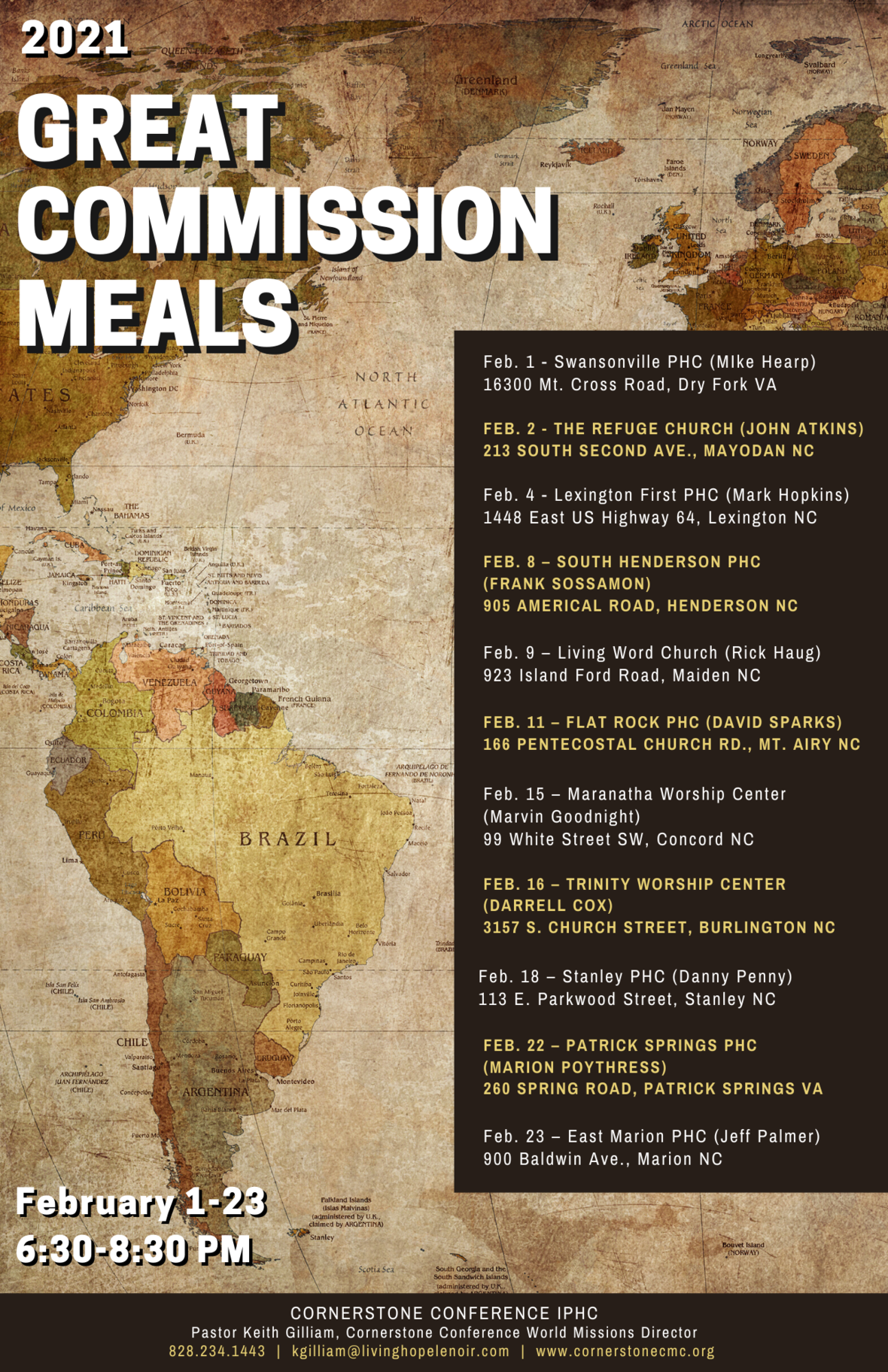# Great Commission Meals

## February 1-23 from 6:30-8:30 PM

Great Commission Meals are a great way to meet Missionaries, learn more about World Mission Ministries, and find out what God is doing in the world. A representative from World Missions Ministries will be present at each meal, to answer any questions you might have.

Come join us and find out how you can be a part of reaching His harvest!

Pastor Keith Gilliam, Cornerstone Conference World Missions Director
kgilliam@livinghopelenoir.com
828.234.1443

 table div table+table+table+table+table+table+table+table+table+table+table+table+table+table+table+table+table+table+table+table div table{width:100%;padding:0}table div table+table+table+table+table+table+table+table+table+table+table+table+table+table+table+table+table+table+table+table div table img{width:96.23%;padding:0;float:none}table div table+table+table+table+table+table+table+table+table+table+table+table+table+table+table+table+table+table+table+table div table td{width:100%;padding:0 1.88% 18px}/* styles */# Big Fish…Small Pond

## By Joel Boyles, Resident Director of Discipleship Ministries

We can all agree that we are happy to see the year 2020 come to an end! Due to the pandemic, we had to cancel many significant events. More importantly, local churches had to develop new strategies to be able to minister to their congregations.

It is my desire that 2021 does not look like 2020. Hopefully 2021 is a much better year, and we will see our lives, churches and events, return to a somewhat normal routine!

As we enter into the new year, there is something I want us to ask ourselves:

How can I become better at discipling people?

We can do this, by being a BIG fish in a small pond!

What does that mean?

Each of us have influence on a small, specific group of people in our lives. The size of your church does not matter! YOU have an influence in the lives of others! There are times we are unaware of how much influence we actually have in the moment. Sometimes many years pass before we are able to see the difference we made in a person's life.

I can remember my Junior Boys Sunday School class. We had 8-10 boys in that class and my uncle, Steele, was our teacher. Not only did he teach us on Sunday mornings, but he poured into us during the week, influencing us in every area of life. One of those ways he influenced us, was taking us to the lake for fishing! Today, two of those Junior boys in that Sunday School class are in full time ministry!

Remember, Discipleship is a life long journey! So, let’s start influencing those who are in our “pond” to grow and be disciples of Jesus Christ!

 table div table+table+table+table+table+table+table+table+table+table+table+table+table+table+table+table+table+table+table+table+table+table+table div table{width:100%;padding:0}table div table+table+table+table+table+table+table+table+table+table+table+table+table+table+table+table+table+table+table+table+table+table+table div table img{width:96.23%;padding:0;float:none}table div table+table+table+table+table+table+table+table+table+table+table+table+table+table+table+table+table+table+table+table+table+table+table div table td{width:100%;padding:0 1.88% 18px}/* styles */table div table+table+table+table+table+table+table+table+table+table+table+table+table+table+table+table+table+table+table+table+table+table+table+table div table td,table.module-23{width:100%;padding:0}table div table+table+table+table+table+table+table+table+table+table+table+table+table+table+table+table+table+table+table+table+table+table+table+table div table{width:100%;float:none;margin-left:auto;margin-right:auto;padding:0}table div table+table+table+table+table+table+table+table+table+table+table+table+table+table+table+table+table+table+table+table+table+table+table+table div table a{border:0 none;text-decoration:none}table div table+table+table+table+table+table+table+table+table+table+table+table+table+table+table+table+table+table+table+table+table+table+table+table div table img{width:100%!important;border:0 none;text-decoration:none}/* styles */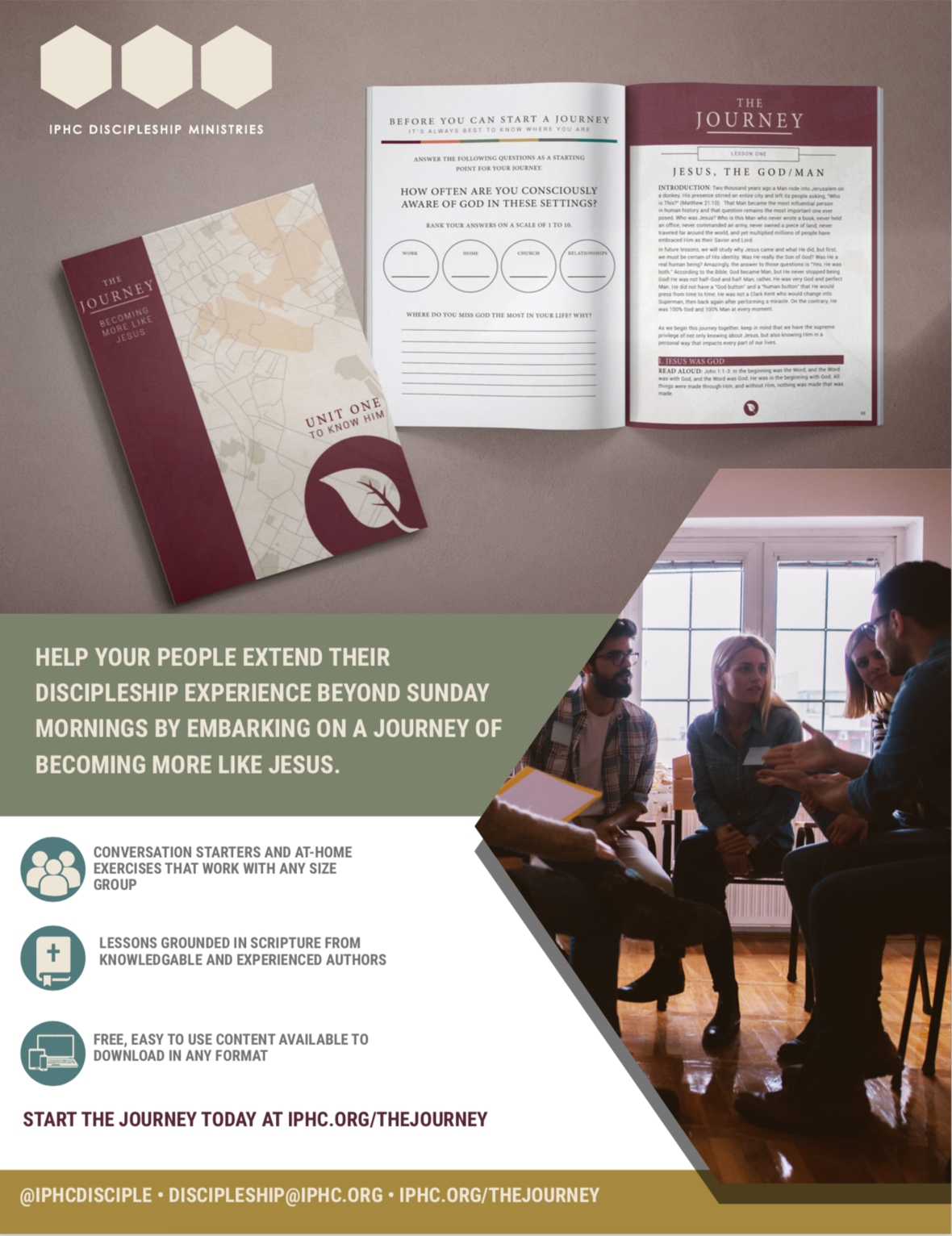table div table+table+table+table+table+table+table+table+table+table+table+table+table+table+table+table+table+table+table+table+table+table+table+table+table+table+table div table{width:100%;padding:0}table div table+table+table+table+table+table+table+table+table+table+table+table+table+table+table+table+table+table+table+table+table+table+table+table+table+table+table div table img{width:96.23%;padding:0;float:none}table div table+table+table+table+table+table+table+table+table+table+table+table+table+table+table+table+table+table+table+table+table+table+table+table+table+table+table div table td{width:100%;padding:0 1.88% 18px}/* styles */# Lift up your eyes and work in partnership

## By Joel J. Pava, Resident Director of Hispanic Ministries

God declares in His Word, that "soul winning" should be important to every Christian: “Go ye into all the world, and preach the gospel to every creature” (Mark 16:15); and “Go ye therefore, and teach all nations, baptizing them in the name of the Father, and of the Son, and of the Holy Ghost” (Matthew 28:19).

Often, we don’t have to travel very far to find those who need Jesus. There are people around us each day that need to hear the Gospel, not just those in other countries. God sent the nations to us, to be able to witness to them who He is, and what He has done for us. All we need to do is to tell others about Jesus, hoping that the new believers will preach the Gospel to their loved ones, whether here in USA or back in their country of origin.

In 2012, citing cartoMission.com, “Over 40 million people living in the United States were born in another country, according to recent figures from the U.S. Census Bureau. The place of birth for these people include over 100 countries from across the globe. Mexico is the birthplace of approximately 28% of the foreign-born population living in the U.S. The next largest populations come from India, Philippines, China, El Salvador, Vietnam, Cuba, Korea, Dominican Republic, and Guatemala. So, in the top 10 countries of birth for immigrants in the U.S., five are from Latin America and five are from Asia."

What a great opportunity God has given us to witness to the nations!

The Bible tells us two things that we need to do to make it happen:

1. “…behold, I say unto you, Lift up your eyes, and look on the fields; for they are white already to harvest.” (John 4:35). There is a big harvest near every Anglo church in USA. That harvest is within those 40 million + immigrants living here. By lifting up our eyes we can see this new harvest and then with God’s guidance and a godly heart we would be able to fill empty pews, chairs and buildings with new converted and renewed ministry.
2. “And they beckoned unto their partners, which were in the other ship, that they should come and help them. And they came, and filled both the ships, so that they began to sink”. (Luke 5:7). We are convinced that the harvest requires partnership. With all the gifts God has given to His church and having those gifts available to serve others, partnership becomes a major ally to obtain the miraculous catch of souls during this time and season.
 1 “…behold, I say unto you, Lift up your eyes, and look on the fields; for they are white already to harvest.” (John 4:35). There is a big harvest near every Anglo church in USA. That harvest is within those 40 million + immigrants living here. By lifting up our eyes we can see this new harvest and then with God’s guidance and a godly heart we would be able to fill empty pews, chairs and buildings with new converted and renewed ministry.
 2 “And they beckoned unto their partners, which were in the other ship, that they should come and help them. And they came, and filled both the ships, so that they began to sink”. (Luke 5:7). We are convinced that the harvest requires partnership. With all the gifts God has given to His church and having those gifts available to serve others, partnership becomes a major ally to obtain the miraculous catch of souls during this time and season.

Lifting up our eyes and working for God’s kingdom in partnership with the migrant population would help us to reach the nations and the new harvest!

 table div table+table+table+table+table+table+table+table+table+table+table+table+table+table+table+table+table+table+table+table+table+table+table+table+table+table+table+table+table+table div table{width:100%;padding:0}table div table+table+table+table+table+table+table+table+table+table+table+table+table+table+table+table+table+table+table+table+table+table+table+table+table+table+table+table+table+table div table img{width:96.23%;padding:0;float:none}table div table+table+table+table+table+table+table+table+table+table+table+table+table+table+table+table+table+table+table+table+table+table+table+table+table+table+table+table+table+table div table td{width:100%;padding:0 1.88% 18px}/* styles */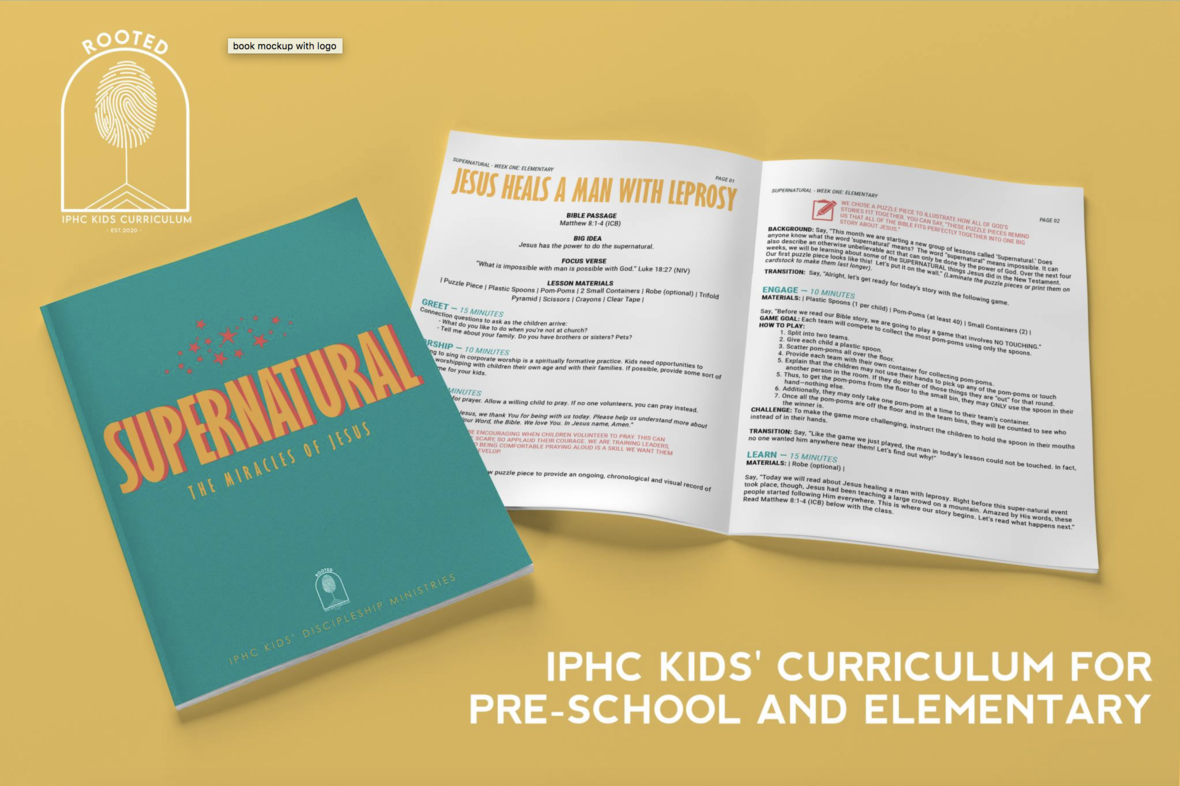# Looking for a Curriculum that will help your kids understand who God is? THIS IS IT!

## New IPHC Kids' Cirriculum "Rooted"

We are proud to offer ROOTED Kids' Curriculum to you. We hope that this IPHC product is a blessing to your church and your children! Below are two resources to help you get the most out of each lesson of ROOTED.

Click on the button below to access all of the Rooted Cirriculum Downloads today!

 table.module-34{width:83.77%;padding:0}table div table+table+table+table+table+table+table+table+table+table+table+table+table+table+table+table+table+table+table+table+table+table+table+table+table+table+table+table+table+table+table+table+table+table+table div table{width:83.77%;float:none;margin-left:auto;margin-right:auto;padding:0}table div table+table+table+table+table+table+table+table+table+table+table+table+table+table+table+table+table+table+table+table+table+table+table+table+table+table+table+table+table+table+table+table+table+table+table div table a{border:0 none;text-decoration:none}table div table+table+table+table+table+table+table+table+table+table+table+table+table+table+table+table+table+table+table+table+table+table+table+table+table+table+table+table+table+table+table+table+table+table+table div table img{width:100%!important;border:0 none;text-decoration:none}table div table+table+table+table+table+table+table+table+table+table+table+table+table+table+table+table+table+table+table+table+table+table+table+table+table+table+table+table+table+table+table+table+table+table+table div table td{width:100%;padding:0}/* styles */
 table.module-35{width:83.96%;padding:0}table div table+table+table+table+table+table+table+table+table+table+table+table+table+table+table+table+table+table+table+table+table+table+table+table+table+table+table+table+table+table+table+table+table+table+table+table div table{width:83.96%;float:none;margin-left:auto;margin-right:auto;padding:0}table div table+table+table+table+table+table+table+table+table+table+table+table+table+table+table+table+table+table+table+table+table+table+table+table+table+table+table+table+table+table+table+table+table+table+table+table div table a{border:0 none;text-decoration:none}table div table+table+table+table+table+table+table+table+table+table+table+table+table+table+table+table+table+table+table+table+table+table+table+table+table+table+table+table+table+table+table+table+table+table+table+table div table img{width:100%!important;border:0 none;text-decoration:none}table div table+table+table+table+table+table+table+table+table+table+table+table+table+table+table+table+table+table+table+table+table+table+table+table+table+table+table+table+table+table+table+table+table+table+table+table div table td{width:100%;padding:0}/* styles */
 table div table+table+table+table+table+table+table+table+table+table+table+table+table+table+table+table+table+table+table+table+table+table+table+table+table+table+table+table+table+table+table+table+table+table+table+table+table div table{width:100%;padding:0}table div table+table+table+table+table+table+table+table+table+table+table+table+table+table+table+table+table+table+table+table+table+table+table+table+table+table+table+table+table+table+table+table+table+table+table+table+table div table img{width:96.23%;padding:0;float:none}table div table+table+table+table+table+table+table+table+table+table+table+table+table+table+table+table+table+table+table+table+table+table+table+table+table+table+table+table+table+table+table+table+table+table+table+table+table div table td{width:100%;padding:0 1.88% 18px}/* styles */# Rooted: IPHC Kids Virtual Conference videos and resources are now available!

Family discipleship is a HUGE topic this year as 2020 still has us navigating online church, church-at-home, and simply more time for kids and parents to be together.

In November, our IPHC Kids Ministry, released their first virtual children's ministry training! Kristi Cain, IPHC Kids Ministries Director, writes:

"We're calling it "Rooted," because, we want our children rooted and established in Christ (Colossians 2:6-7). We want them to grow into "trees of righteousness" (Isaiah 61:1-3) for the glory of our Lord."

If you missed our Virtual Rooted Conference, you can find all of the videos and resources online at www.iphc.org/discipleship/rooted or by clicking on the links below.

 table.module-40{width:86.42%;padding:0}table div table+table+table+table+table+table+table+table+table+table+table+table+table+table+table+table+table+table+table+table+table+table+table+table+table+table+table+table+table+table+table+table+table+table+table+table+table+table+table+table+table div table{width:86.42%;float:none;margin-left:auto;margin-right:auto;padding:0}table div table+table+table+table+table+table+table+table+table+table+table+table+table+table+table+table+table+table+table+table+table+table+table+table+table+table+table+table+table+table+table+table+table+table+table+table+table+table+table+table+table div table a{border:0 none;text-decoration:none}table div table+table+table+table+table+table+table+table+table+table+table+table+table+table+table+table+table+table+table+table+table+table+table+table+table+table+table+table+table+table+table+table+table+table+table+table+table+table+table+table+table div table img{width:100%!important;border:0 none;text-decoration:none}table div table+table+table+table+table+table+table+table+table+table+table+table+table+table+table+table+table+table+table+table+table+table+table+table+table+table+table+table+table+table+table+table+table+table+table+table+table+table+table+table+table div table td{width:100%;padding:0}/* styles */
 table div table+table+table+table+table+table+table+table+table+table+table+table+table+table+table+table+table+table+table+table+table+table+table+table+table+table+table+table+table+table+table+table+table+table+table+table+table+table+table+table+table+table div table{width:100%;padding:0}table div table+table+table+table+table+table+table+table+table+table+table+table+table+table+table+table+table+table+table+table+table+table+table+table+table+table+table+table+table+table+table+table+table+table+table+table+table+table+table+table+table+table div table table{padding:0;float:left!important;width:34.934%!important}table div table+table+table+table+table+table+table+table+table+table+table+table+table+table+table+table+table+table+table+table+table+table+table+table+table+table+table+table+table+table+table+table+table+table+table+table+table+table+table+table+table+table div table td{padding-left:36px;padding-right:36px}table div table+table+table+table+table+table+table+table+table+table+table+table+table+table+table+table+table+table+table+table+table+table+table+table+table+table+table+table+table+table+table+table+table+table+table+table+table+table+table+table+table+table div table table td,table div table+table+table+table+table+table+table+table+table+table+table+table+table+table+table+table+table+table+table+table+table+table+table+table+table+table+table+table+table+table+table+table+table+table+table+table+table+table+table+table+table+table div table table+table td{padding-left:0;padding-right:20px}table div table+table+table+table+table+table+table+table+table+table+table+table+table+table+table+table+table+table+table+table+table+table+table+table+table+table+table+table+table+table+table+table+table+table+table+table+table+table+table+table+table+table div table table+table{float:left!important;width:34.716%!important}table div table+table+table+table+table+table+table+table+table+table+table+table+table+table+table+table+table+table+table+table+table+table+table+table+table+table+table+table+table+table+table+table+table+table+table+table+table+table+table+table+table+table div table table+table+table{float:left!important;width:30.349999999999994%!important}table div table+table+table+table+table+table+table+table+table+table+table+table+table+table+table+table+table+table+table+table+table+table+table+table+table+table+table+table+table+table+table+table+table+table+table+table+table+table+table+table+table+table div table table+table+table td{padding-left:0;padding-right:0}/* styles */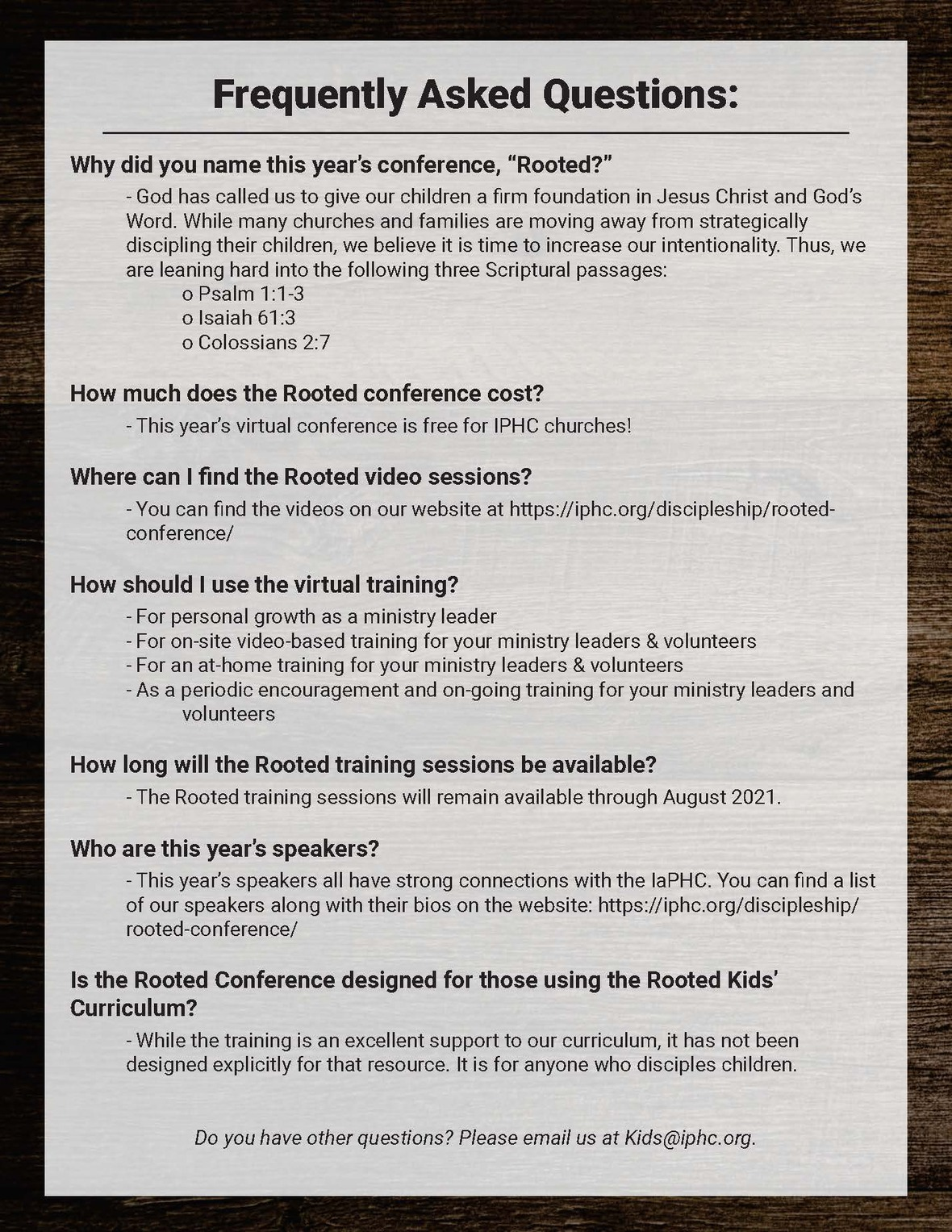table div table+table+table+table+table+table+table+table+table+table+table+table+table+table+table+table+table+table+table+table+table+table+table+table+table+table+table+table+table+table+table+table+table+table+table+table+table+table+table+table+table+table+table+table div table{width:100%;padding:0}table div table+table+table+table+table+table+table+table+table+table+table+table+table+table+table+table+table+table+table+table+table+table+table+table+table+table+table+table+table+table+table+table+table+table+table+table+table+table+table+table+table+table+table+table div table img{width:96.23%;padding:0;float:none}table div table+table+table+table+table+table+table+table+table+table+table+table+table+table+table+table+table+table+table+table+table+table+table+table+table+table+table+table+table+table+table+table+table+table+table+table+table+table+table+table+table+table+table+table div table td{width:100%;padding:0 1.88% 18px}/* styles */table div table+table+table+table+table+table+table+table+table+table+table+table+table+table+table+table+table+table+table+table+table+table+table+table+table+table+table+table+table+table+table+table+table+table+table+table+table+table+table+table+table+table+table+table+table div table td,table.module-44{width:100%;padding:0}table div table+table+table+table+table+table+table+table+table+table+table+table+table+table+table+table+table+table+table+table+table+table+table+table+table+table+table+table+table+table+table+table+table+table+table+table+table+table+table+table+table+table+table+table+table div table{width:100%;float:none;margin-left:auto;margin-right:auto;padding:0}table div table+table+table+table+table+table+table+table+table+table+table+table+table+table+table+table+table+table+table+table+table+table+table+table+table+table+table+table+table+table+table+table+table+table+table+table+table+table+table+table+table+table+table+table+table div table a{border:0 none;text-decoration:none}table div table+table+table+table+table+table+table+table+table+table+table+table+table+table+table+table+table+table+table+table+table+table+table+table+table+table+table+table+table+table+table+table+table+table+table+table+table+table+table+table+table+table+table+table+table div table img{width:100%!important;border:0 none;text-decoration:none}/* styles */

# New eBook Available: Leading a Post-COVID Church

## By Jonathan Hill, Resident Director of Evangelism Ministries

Probably most of us thought Covid-19 was going to be a challenge for a few months, max. We now know nothing could be further from the truth. As we enter into a new year, we are still facing new challenges of how to navigate our local churches during perplexing times.

Dr. Sam Rainer has assembled an e-book called “Leading A Post-Covid Church.” You will find some helpful awareness Sam has gathered from thousands of ministers across the United States and around the world, who share their experiences on a platform known as Church Answers.

This site has been very insightful for me to hear from local pastors of various backgrounds, and the challenges and successes they are experiencing. Please take a few minutes to peruse this 30-page document, and hopefully it will help you process some of the next steps for “being the church.”

 table div table+table+table+table+table+table+table+table+table+table+table+table+table+table+table+table+table+table+table+table+table+table+table+table+table+table+table+table+table+table+table+table+table+table+table+table+table+table+table+table+table+table+table+table+table+table+table+table div table{width:100%;padding:0}table div table+table+table+table+table+table+table+table+table+table+table+table+table+table+table+table+table+table+table+table+table+table+table+table+table+table+table+table+table+table+table+table+table+table+table+table+table+table+table+table+table+table+table+table+table+table+table+table div table img{width:96.23%;padding:0;float:none}table div table+table+table+table+table+table+table+table+table+table+table+table+table+table+table+table+table+table+table+table+table+table+table+table+table+table+table+table+table+table+table+table+table+table+table+table+table+table+table+table+table+table+table+table+table+table+table+table div table td{width:100%;padding:0 1.88% 18px}/* styles */# Upcoming Online BMI Classes

January 8-9 Class - Eschatology
Instructor: Danny Penny
Text: Be Victorious by Warren W. Wiersbe

February 5-6 Class - Church Growth
Instructor: Anthony Craver
Text: Lasting Impact by Carey Nieuwhof

 table div table+table+table+table+table+table+table+table+table+table+table+table+table+table+table+table+table+table+table+table+table+table+table+table+table+table+table+table+table+table+table+table+table+table+table+table+table+table+table+table+table+table+table+table+table+table+table+table+table+table+table div table{width:100%;padding:0}table div table+table+table+table+table+table+table+table+table+table+table+table+table+table+table+table+table+table+table+table+table+table+table+table+table+table+table+table+table+table+table+table+table+table+table+table+table+table+table+table+table+table+table+table+table+table+table+table+table+table+table div table img{width:96.23%;padding:0;float:none}table div table+table+table+table+table+table+table+table+table+table+table+table+table+table+table+table+table+table+table+table+table+table+table+table+table+table+table+table+table+table+table+table+table+table+table+table+table+table+table+table+table+table+table+table+table+table+table+table+table+table+table div table td{width:100%;padding:0 1.88% 18px}/* styles */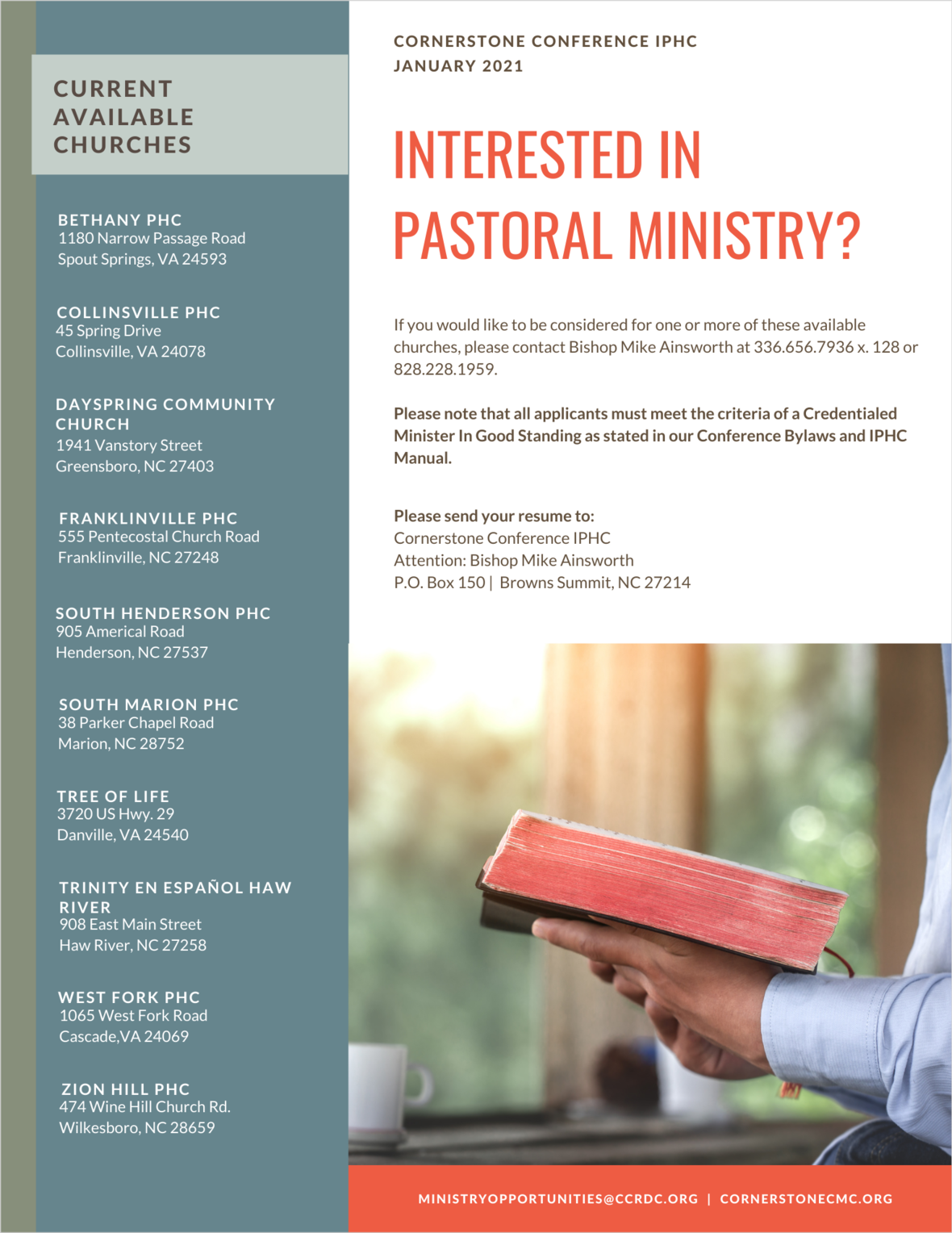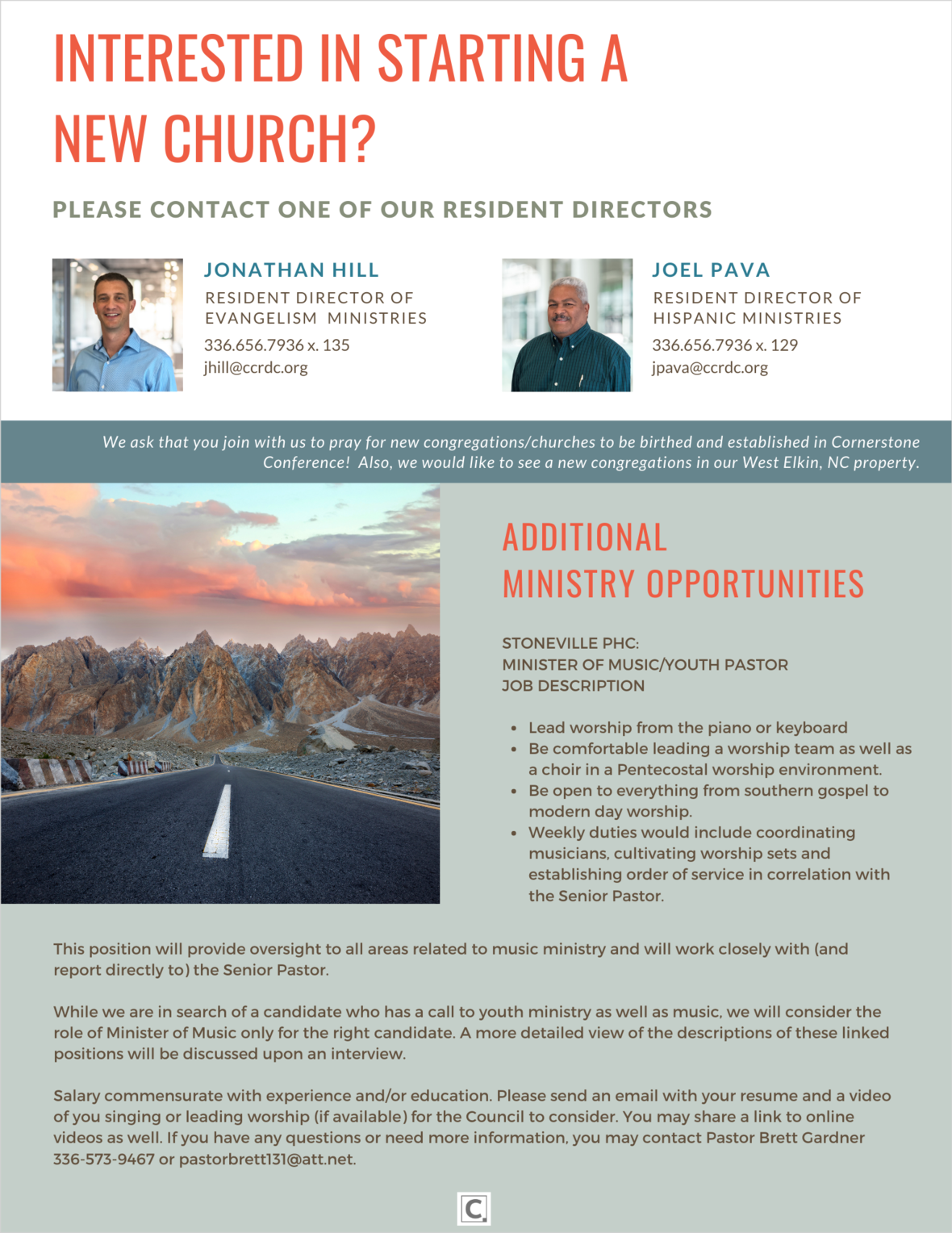table div table+table+table+table+table+table+table+table+table+table+table+table+table+table+table+table+table+table+table+table+table+table+table+table+table+table+table+table+table+table+table+table+table+table+table+table+table+table+table+table+table+table+table+table+table+table+table+table+table+table+table+table+table+table+table div table{width:100%;padding:0}table div table+table+table+table+table+table+table+table+table+table+table+table+table+table+table+table+table+table+table+table+table+table+table+table+table+table+table+table+table+table+table+table+table+table+table+table+table+table+table+table+table+table+table+table+table+table+table+table+table+table+table+table+table+table+table div table img{width:96.23%;padding:0;float:none}table div table+table+table+table+table+table+table+table+table+table+table+table+table+table+table+table+table+table+table+table+table+table+table+table+table+table+table+table+table+table+table+table+table+table+table+table+table+table+table+table+table+table+table+table+table+table+table+table+table+table+table+table+table+table+table div table td{width:100%;padding:0 1.88% 18px}/* styles */